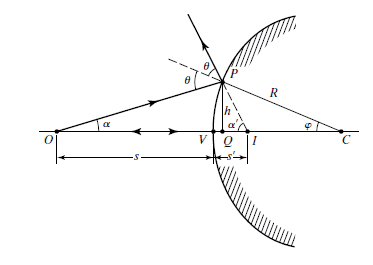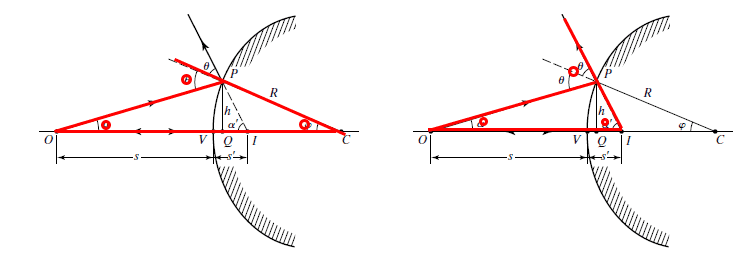Geometrical Optics - Light ray angles on a spherical mirrorI can't see how the textbook produces the following relationships between angles:
$$\theta = \phi + \alpha \qquad (1)$$
$$2\theta = \alpha + \alpha ' \qquad (2)$$

My thinking is that the exterior angle theorem for triangles was used to create expression ##(1)##, but I am unsure as to how expression ##(2)## came about. Was the exterior angle theorem simply used twice to produce both equations?

Attachments